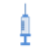canSAR2276585
FEATURESNAMES
SMILES
CCCC[C@@H]1NC(=O)[C@H](CCC(N)=O)NC(=O)[C@H](CCCNC(=N)N)NC(=O)[C@@H]2CSCCC(=O)N3CN(CN(C3)C(=O)CCSC[C@@H](C(=O)N[C@@H](CCCNC(=N)N)C(=O)N[C@@H](Cc3c[nH]c4ccccc34)C(=O)O)NC(=O)[C@H](CCCNC(=N)N)NC1=O)C(=O)CCSC[C@H](NC(=O)[C@@H](N)CCCNC(=N)N)C(=O)N[C@@H](Cc1ccccc1)C(=O)N[C@@H](CCCNC(=N)N)C(=O)N[C@@H](CC(C)C)C(=O)N1CCC[C@H]1C(=O)N2
InChI
MOLECULAR FORMULA
C93H148N34O19S3
CROSS REFERENCES# canSAR2276585

## Compound Synopsis

OVERVIEW
PROPERTIES
Molecular weight 2141.08
AlogP -6.24
HBond donors 38
HBond acceptors 53
Atoms 297
Contains toxicophore No
Contains PAINS No
Commercially available Unknown
Property color indications are based on Lipinski's Ro5.
PROTEIN TARGETS
No information about proteins targeted by canSAR2276585.